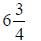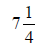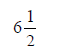# CBSE Class 5 Mathematics Average MCQs

## MCQ for Class 5 Mathematics Average

Class 5 Mathematics students should refer to the following multiple-choice questions with answers for Average in standard 5. These MCQ questions with answers for Grade 5 Mathematics will come in exams and help you to score good marks

### Average MCQ Questions Class 5 Mathematics with Answers

Question: The average of first 5 odd number is

a) 5

b) 6

c) 4

Question: The average of first five counting number is

a) 3

b) 4

c) 5

Question: The average of first five multiples of 4 is

a) 12

b) 6

c) 3

Question: The average of first four composite number is

a)b)c)Question: Average of 3, 4 &5 = ___________

a) 4

b) 5

c) 6

Question: Average of 37, 38, 45, 50 =

a) 42.5

b) 42

c) 50

Question: Average of 5, 7, 0, 8 = ___________

a) 5

b) 4

c) 3

Question: Average of 6, 14, 20, 32, 0, 11, 8 =

a) 13

b) 14

c) 15

Question: Average of 8 and 10 =

a) 9

b) 10

c) 11

Question: The average of first five multiples of 3 is

a) 9

b) 10

c) 8

Question: The average of first five multiples of 5 is

a) 15

b) 14

c) 13

Question: Riya obtained 65, 67, 76, 82 and 50 out of 100 in different subjects. What will be the average?

a) 68

b) 76

c) 75

Question: Find the average of first 10 multiples of 7

a) 38.5

b) 40.5

c) 37.5

Question: The average of first five multiples of 9 is ___________

a) 27

b) 28

c) 20

Question: Average of 3.6, 5.9, 9.7, 8.3, 1.2, 4.6 =

a) 5.55

b) 13

c) 14

Question: Average of 6, 14, 20,32, 0, 11, 8 =

a) 13

b) 14

c) 12

Question: Average of 50, 55, 48 = _

a) 51

b) 52

c) 53

Question: Average of 45, 45, 44, 44, 43, 43 =

a) 44

b) 45

c) 35

Question: Average of first 5 multiples of 10 =

a) 30

b) 25

c) 70

Question: Average of 3 multiples of 12 =

a) 24

b) 25

c) 20

Question: Average of first 5 multiples of 15 =

a) 45

b) 55

c) 65

Question: Average of first 3 multiples of 13 =

a) 26

b) 22

c) 20

Question: Average of first 5 multiples of 9 =

a) 27

b) 28

c) 26

Question: Average of first 3 multiples of 14 =

a) 28

b) 35

c) 25